# Wiedemann-Franz Law

(redirected from Wiedemann franz law)

## Wiedemann-Franz law

An empirical law of physics which states that the ratio of the thermal conductivity of a metal to its electrical conductivity is a constant times the absolute temperature, as given by the equation below. Here Kc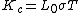is the thermal conductivity due to the conduction electrons, σ is the electrical conductivity, T is the absolute temperature, and L0 is known as the Lorentz number. The Wiedemann-Franz law provides an important check on theories of electrical and thermal conductivity. See Conduction (heat), Thermal conduction in solids

McGraw-Hill Concise Encyclopedia of Physics. © 2002 by The McGraw-Hill Companies, Inc.
The following article is from The Great Soviet Encyclopedia (1979). It might be outdated or ideologically biased.

## Wiedemann-Franz Law

the correlation between the heat conductivity and electrical conductivity of metals.

In 1853, G. Wiedemann and R. Franz (1827-1902) established on the basis of experimental data that for all metals the ratio of heat conductivity K to electrical conductivity σ at a constant temperature is the same: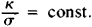In 1882 the Danish physicist L. Lorenz demonstrated that the relationship k/σ changes in direct proportion to the abso-lute temperature T: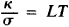where L is a constant that is identical for all metals; this is called the Lorenz number.

The interconnection between electrical conductivity and heat conductivity is explained by the fact that both these properties of metals are mainly caused by the movement of electrons. The Wiedemann-Franz law was explained for the first time by the German physicist P. Drude, who regarded electrons in metal as a gas and who applied to them the methods of the classical kinetic theory of gases. However, it was only with the aid of quantum statistics that an expression was obtained for L that was in good agreement with the experiment: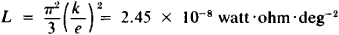where k is the Boltzmann constant and e is the charge of an electron. However, the Wiedemann-Franz law is consistent with experiment only within a fixed interval of temperatures (see Figure 1).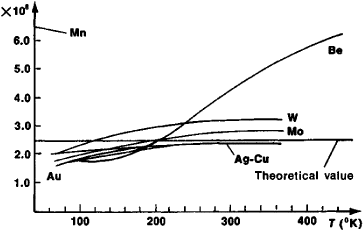Figure 1. Dependence of the Lorenz number, L X 108, on temperature T for certain metals

Present-day solid-state theory, which takes into consideration the interaction of electrons with crystal-lattice vibrations (electron-phonon interaction), explains not only the Wiedemann-Franz law but also the cause of deviations from it. At room temperature the observed values of L are fully consistent with its theoretical values. However, there are metals (for example, Be), which at room temperature are not subject to the Wiedemann-Franz law. The reason for this is apparently the large value of the Debye temperature for these metals.